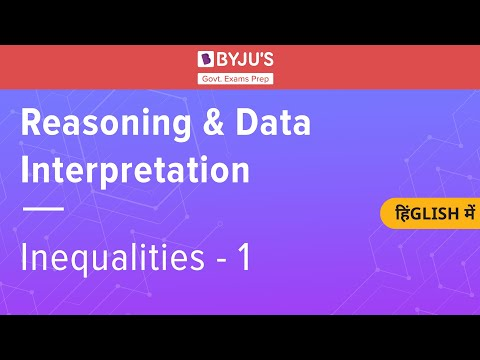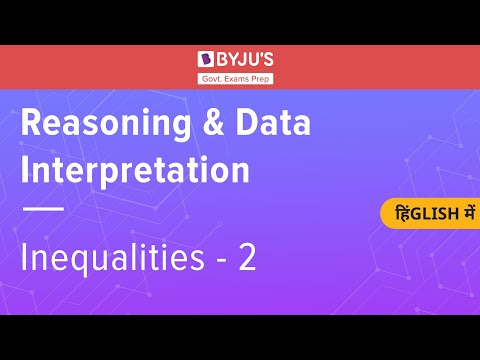# Reasoning Inequality Questions & Answers

The reasoning inequality questions along with their answers have been given in this article for candidates to prepare themselves and master the topic.

It is one of the most common logical reasoning topics and candidates preparing for Bank, Insurance and Government exams must practise more and more questions based on this concept.

 Ace the Reasoning Ability section for the upcoming Government exams with the links given below:

Furthermore, candidates aspiring to join the Government sector must know that with the increase in the number of applicants, ensuring that they prepare every topic under each subject equally well is extremely important.

Apart from the logical reasoning section, candidates can also visit the articles given below to strengthen their preparation and improve their performance in the other sections of the examination:

## Reasoning Inequality Questions

Candidates who are not familiar with the concept of reasoning inequality can visit the linked article to know more about the topic and some simple tips and tricks to answer questions based on it.

Solving the inequality questions given below will boost the confidence of a candidate and also enable them to solve questions within a shorter time span.

Before heading to solve the questions, candidates must go through the video on reasoning inequalities given below to clarify the tips and strategies to follow for taking up questions in the examination.Directions (Q1 – Q5): In each of the given questions, one statement has been given followed by two conclusions. Find which of the given conclusions is true

Q 1.

Statement: A > F ≤ C = D < E

Conclusion I: A > E

Conclusion II: F < E

1. Only conclusion I is true
2. Only conclusion II is true
3. Both conclusion I and II are true
4. Neither conclusion I nor II is true
5. Either conclusion I or II is true

Q 2.

Statement: P > Q, X ≤ R < S, S > P

Conclusion I: P ≤ R

Conclusion II: X > S

1. Only conclusion I is true
2. Only conclusion II is true
3. Both conclusion I and II are true
4. Neither conclusion I nor II is true
5. Either conclusion I or II is true

Q 3.

Statement: V ≤ X > Y ≤ U = Z > O

Conclusion I: Y < Z

Conclusion II: Y = Z

1. Only conclusion I is true
2. Only conclusion II is true
3. Both conclusion I and II are true
4. Neither conclusion I nor II is true
5. Either conclusion I or II is true

Q 4.

Statement: C = B ≥ A ≤ D = E

Conclusion I: C = X

Conclusion II: C < D

1. Only conclusion I is true
2. Only conclusion II is true
3. Both conclusion I and II are true
4. Neither conclusion I nor II is true
5. Either conclusion I or II is true

Q 5.

Statement: L > M, M ≤ O = N, L = Q < K

Conclusion I: K > M

Conclusion II: O ≤ K

1. Only conclusion I is true
2. Only conclusion II is true
3. Both conclusion I and II are true
4. Neither conclusion I nor II is true
5. Either conclusion I or II is trueDirections (Q6 – Q10):  In the following questions, the symbols #, *, %, @ and © are used with the following meaning:

A # B, means A is greater than B

A * B, means A is smaller than B

A % B, means A is equal to B

A @ B, means A is greater than equal to B

A © B, means A is smaller than equal to B

Q 6.

Statement: S © P @ Q # R

Conclusion I: S @ R

Conclusion II: R * P

1. Only conclusion I is true
2. Only conclusion II is true
3. Both conclusion I and II are true
4. Neither conclusion I nor II is true
5. Either conclusion I or II is true

Q 7.

Statement: X # B * N @ I © H

Conclusion I: X # N

Conclusion II: I % X

1. Only conclusion I is true
2. Only conclusion II is true
3. Both conclusion I and II are true
4. Neither conclusion I nor II is true
5. Either conclusion I or II is true

Q 8.

Statement: A % R © U @ D % G

Conclusion I: A % U

Conclusion II: A * U

1. Only conclusion I is true
2. Only conclusion II is true
3. Both conclusion I and II are true
4. Neither conclusion I nor II is true
5. Either conclusion I or II is true

Q 9.

Statement: H % J * D * K # I % F

Conclusion I: J * K

Conclusion II: D % F

1. Only conclusion I is true
2. Only conclusion II is true
3. Both conclusion I and II are true
4. Neither conclusion I nor II is true
5. Either conclusion I or II is true

Q 10.

Statement: L # H % J % B @ D # F

Conclusion I: H % F

Conclusion II: L # D

1. Only conclusion I is true
2. Only conclusion II is true
3. Both conclusion I and II are true
4. Neither conclusion I nor II is true
5. Either conclusion I or II is true

Q 11.

Statement: K < H > G, G ≤ N, N = U

Conclusion I: K = U

Conclusion II: H > N

1. Only conclusion I is true
2. Only conclusion II is true
3. Both conclusion I and II are true
4. Neither conclusion I nor II is true
5. Either conclusion I or II is true

Q 12.

Statement: G ≤ S, S > R < K, K ≥ C, L = G

Conclusion I: G ≤ R

Conclusion II: L ≥ K

1. Only conclusion I is true
2. Only conclusion II is true
3. Both conclusion I and II are true
4. Neither conclusion I nor II is true
5. Either conclusion I or II is true

Direction (Q13 – Q15): Based on the information given below, answer the following questions:

A @ B, means B is greater than A

A & B, means B is smaller than A

A $B, means B is equal to A A # B, means B is greater than equal to A A % B, means B is smaller than or equal to A Q 13. Statement: P @ Q$ R % S # T % U

Conclusion I: P % U

Conclusion II: R @ T

1. Only conclusion I is true
2. Only conclusion II is true
3. Both conclusion I and II are true
4. Neither conclusion I nor II is true
5. Either conclusion I or II is true

Q 14.

Statement: A @ B $C & D @ E # F Conclusion I: F @ C Conclusion II: A # D 1. Only conclusion I is true 2. Only conclusion II is true 3. Both conclusion I and II are true 4. Neither conclusion I nor II is true 5. Either conclusion I or II is true Q 15. Statement: S % U @ T & V$ W # R

Conclusion I: U & R

Conclusion II: T & W

1. Only conclusion I is true
2. Only conclusion II is true
3. Both conclusion I and II are true
4. Neither conclusion I nor II is true
5. Either conclusion I or II is true

Candidates can check the answers to all the inequality questions given above, in the PDF below: